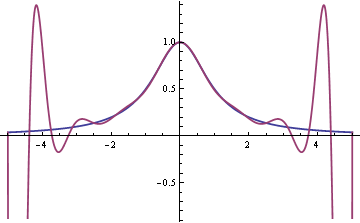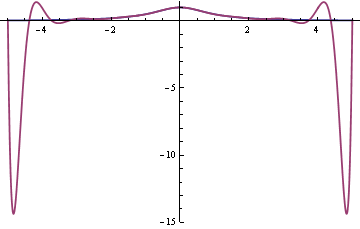# Interpolation errors

Say you have a function f(x) and you want to find a polynomial p(x) that agrees with f(x) at several points. Given a set of points x0, x1, x2, … xn you can always find a polynomial of degree n such that p(xi) = f(xi) for i = 0, 1, 2, …, n. It seems reasonable that the more points you pick, the better the interpolating polynomial p(x) will match the function f(x). If the two functions match exactly at a lot of points, they should match well everywhere. Sometimes that’s true and sometimes it’s not.

Here is a famous example due to Carle Runge. Let f(x) = 1/(1 + x2) and let pn be the polynomial that interpolates f(x) at n+1 evenly spaced nodes in the interval [-5, 5]. As n becomes larger, the fit becomes worse.

Here’s a graph of f(x) and p9(x). The smooth blue line is f(x) and the wiggly red line is p9(x).Here’s the analogous graph for p16(x).The fit is improving in the middle. In fact, the curves agree to within the thickness of the plot line from say -1 to 1. But the fit is so bad in the tails that the graph had to be cut off. Here’s another plot of f(x) and p16(x) on a different scale to show how far negative the polynomial dips.The problem is the spacing of the nodes. Interpolation errors are bad for evenly spaced nodes.

Update: This post explains in a little more depth why this particular function has problems and gives another example where interpolation at evenly-spaced nodes behaves badly.

If we interpolate f(x) at different points, at the Chebyshev nodes, then the fit is good.The Chebyshev nodes on [-1, 1] are xi = cos( π i / n ). Here we multiplied these nodes by 5 to scale to the interval [-5, 5].

If the function f(x) is absolutely continuous, as in our example, then the interpolating polynomials converge uniformly when you interpolate at Chebyshev nodes. However, ordinary continuity is not enough. Given any sequence of nodes, there exists a continuous function such that the polynomial interpolation error grows like log(n) as the number of nodes n increases.

Some numerical integration methods are based on interpolating polynomials: fit a polynomial to the integrand, then integrate the polynomial exactly to approximate the original integral. The examples above suggest that increasing the order of such integration methods might not improve accuracy and might even make things worse.

Related: Need help with interpolation?

## 12 thoughts on “Interpolation errors”

1. My God man. I don’t know how you keep up this quality blogging but I sincerely hope that you are able to continue it for years.

This is by far my favourite blog on the Internet.

2. EastwoodDC

I have encountered this sort of error using the normal CDF function, which is often implemented with a polynomial approximation. The error is not noticeable unless you look at the extreme left tail, where for practical purposes it should evaluate to (essentially) zero.
A quick check shows me that Excel 2007 does not have this problem, or at least the error is so small I can’t easily detect it by looking the PDF/CDF ratio.

3. Daniel Lemire

In Machine Learning or statistics, this is known as overfitting.

What is the lesson here? Always try the simplest possible model that will do the job.

(This is a deep lesson for those doing actual work.)

4. ekzept

@Daniel Lemire,

It can be more than “overfitting”. For example, the satisfactory solution to designing a satisfactory low-pass filter in frequency space not only demands a change in polynomials, it requires a change in norm for measuring deviations. This is the FIR filter regimen, with its equiripple deviations and infinity norm, defeating the problem of “Gibbs towers” which result from using Fourier polynomials. There may be a correspondence between norms and different sampling points or polynomials, but that’s too deep for me to see personally if there is. I know Chebyshev are involved in equiripple.

5. Ana Lemos

With n+1 distinct interpolation nodes we can find a unique polynomial of degree LESS OR EQUAL THAN n interpolating the points (x_i,f(x_i)), i=0,1,…, n.

6. Are the Chebyshev nodes the BEST choice or merely a good choice? How hard is the proof that they are good points?

Cheers,
Mike

7. Tchebychev nodes are really interesting.

8. Jan Galkowski

Similar Gibbs effects afflict Fourier transforms when applied to non-stationary or non-periodic time series. This is one of the reason why some of the wavelet transforms are more attractive for analysis of time series.

9. For the Maclaurin polynomials for 1/(1+x^2), which are p_n(x) = \sum{k=0}^{n} (-x^2)^k, the fit will become better for |x| 1, when n increases.

10. Wavelets have multiple desirable properties. Along with their resistance to the Gibbs effect, they’re computationally attractive: lots of shifts and adds.